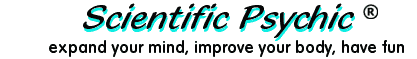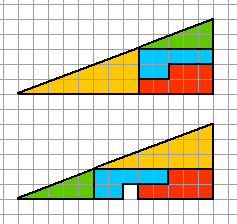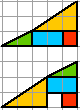Index# The Bermuda Triangle and the Mysterious Square

The Bermuda Triangle is a place in the Atlantic Ocean where ships and airplanes supposedly disappear without a trace. In the picture below, a square appears when we rearrange the pieces of the upper triangle to form the lower triangle. The pieces in both pictures are identical. Can you explain the origin of the square? You will need your knowledge of geometry to solve this problem.### Answer:

The area of a right triangle is computed by multiplying the base times the height and dividing by two. The pieces of the puzzle have an area of 32 square units. Although they can be assembled to form what appears to be a 13×5 right triangle, they actually form quadrilaterals that are slightly smaller or slightly bigger than a 13×5 right triangle. The long side of the these "triangles", i.e, what seems to be the hypotenuse, is not a straight line.

The top figure has an area of 32 square units. The bottom figure, including the empty square, has an area of 33 square units. A real 13×5 right triangle would have an area of 32.5 square units. The distortion is difficult to see because one square of the picture is approximately 3% of the area.

The distortion can be seen more clearly when the empty square constitutes a larger percentage of the area, as in the figure below where 1 square represents 13% of the area.© Copyright  - Antonio Zamora